cancel
Showing results for
Did you mean:New Member

## Line Chart: Average of all Selected Lines during timestamp

Hello,

I tried to keep an average of all selected lines (during each timestamp) on my line chart. However, I don't think PowerBI allows me to keep this curve due to the way legends interact with the data. See below what I mean:

With the legend: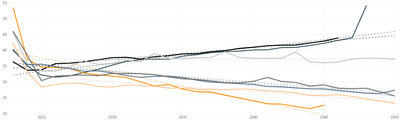Without the legend: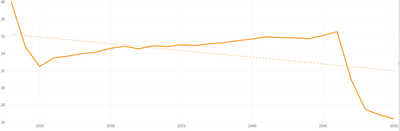In effect, I want the bottom curve (without the legend) overlayed with the top image's curves. Note, the curve without the legend will shift when I select/deselect the various legend values. I want this line to overlay with all unique legend values, and average the selected legend values when specified.

Hope this helps describe my issue in enough depth.

1 ACCEPTED SOLUTIONCommunity Support

Hi @josh122398 ,

Here is my sample:First create a new table like this:

``````let
Source = Table.FromRows(Json.Document(Binary.Decompress(Binary.FromText("Vc6xDcAwCETRXahdGDBJ2iRjWOy/hk1ko0txzZO+oHeSKkKF7jmu5CXlCTlQ3jmxLboqZZSo5CdRcUpblTWUqFRQorK8bvvDE+WrGkpUepH7AA==", BinaryEncoding.Base64), Compression.Deflate)), let _t = ((type nullable text) meta [Serialized.Text = true]) in type table [Year = _t, Legend = _t, Value = _t]),
#"Changed Type" = Table.TransformColumnTypes(Source,{{"Year", Int64.Type}, {"Legend", type text}, {"Value", Int64.Type}}),
#"Removed Other Columns" = Table.SelectColumns(#"Changed Type",{"Legend"}),
#"Removed Duplicates" = Table.Distinct(#"Removed Other Columns"),
#"Expanded Custom" = Table.ExpandListColumn(#"Added Custom", "Custom")
in
#"Expanded Custom"``````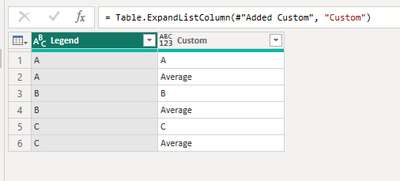Then build relationship between the two tables: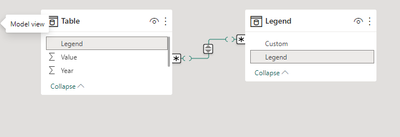Create a slicer:Apply the measure to the line chart:

``````Measure =
VAR _a =
ALLSELECTED ( 'Table'[Legend] )
RETURN
IF (
SELECTEDVALUE ( Legend[Custom] ) = "Average",
CALCULATE (
AVERAGE ( 'Table'[Value] ),
FILTER (
ALL ( 'Table' ),
[Legend]
IN _a
&& [Year] = SELECTEDVALUE ( 'Table'[Year] )
)
),
SUM ( 'Table'[Value] )
)
Measure =
VAR _a =
ALLSELECTED ( 'Table'[Legend] )
RETURN
IF (
SELECTEDVALUE ( Legend[Custom] ) = "Average",
CALCULATE (
AVERAGE ( 'Table'[Value] ),
FILTER (
ALL ( 'Table' ),
[Legend]
IN _a
&& [Year] = SELECTEDVALUE ( 'Table'[Year] )
)
),
SUM ( 'Table'[Value] )
)
``````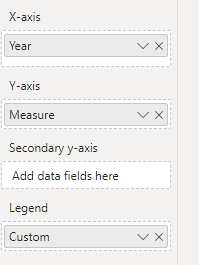Final output:Best Regards,

Jianbo Li

If this post helps, then please consider Accept it as the solution to help the other members find it more quickly.

2 REPLIES 2Community Support

Hi @josh122398 ,

Here is my sample:First create a new table like this:

``````let
Source = Table.FromRows(Json.Document(Binary.Decompress(Binary.FromText("Vc6xDcAwCETRXahdGDBJ2iRjWOy/hk1ko0txzZO+oHeSKkKF7jmu5CXlCTlQ3jmxLboqZZSo5CdRcUpblTWUqFRQorK8bvvDE+WrGkpUepH7AA==", BinaryEncoding.Base64), Compression.Deflate)), let _t = ((type nullable text) meta [Serialized.Text = true]) in type table [Year = _t, Legend = _t, Value = _t]),
#"Changed Type" = Table.TransformColumnTypes(Source,{{"Year", Int64.Type}, {"Legend", type text}, {"Value", Int64.Type}}),
#"Removed Other Columns" = Table.SelectColumns(#"Changed Type",{"Legend"}),
#"Removed Duplicates" = Table.Distinct(#"Removed Other Columns"),
#"Expanded Custom" = Table.ExpandListColumn(#"Added Custom", "Custom")
in
#"Expanded Custom"``````Then build relationship between the two tables:Create a slicer:Apply the measure to the line chart:

``````Measure =
VAR _a =
ALLSELECTED ( 'Table'[Legend] )
RETURN
IF (
SELECTEDVALUE ( Legend[Custom] ) = "Average",
CALCULATE (
AVERAGE ( 'Table'[Value] ),
FILTER (
ALL ( 'Table' ),
[Legend]
IN _a
&& [Year] = SELECTEDVALUE ( 'Table'[Year] )
)
),
SUM ( 'Table'[Value] )
)
Measure =
VAR _a =
ALLSELECTED ( 'Table'[Legend] )
RETURN
IF (
SELECTEDVALUE ( Legend[Custom] ) = "Average",
CALCULATE (
AVERAGE ( 'Table'[Value] ),
FILTER (
ALL ( 'Table' ),
[Legend]
IN _a
&& [Year] = SELECTEDVALUE ( 'Table'[Year] )
)
),
SUM ( 'Table'[Value] )
)
``````Final output:Best Regards,

Jianbo Li

If this post helps, then please consider Accept it as the solution to help the other members find it more quickly.Super User

If this is important to you please consider voting for an existing idea or raising a new one at https://ideas.powerbi.com